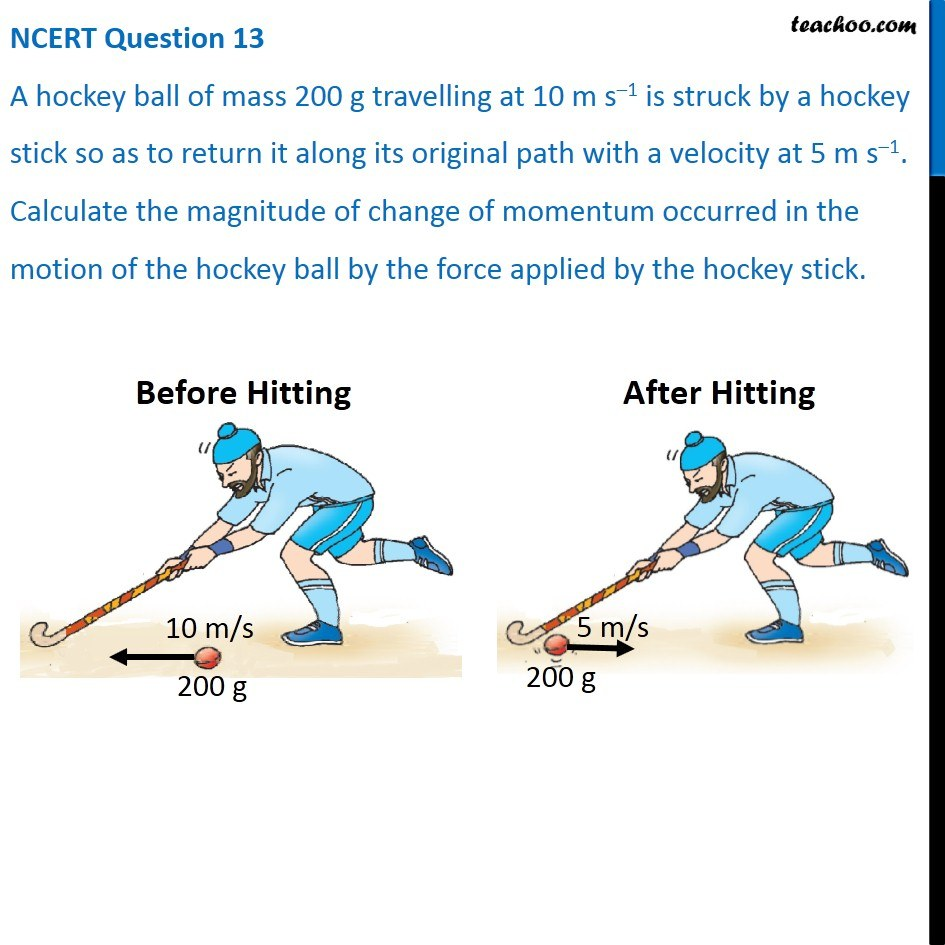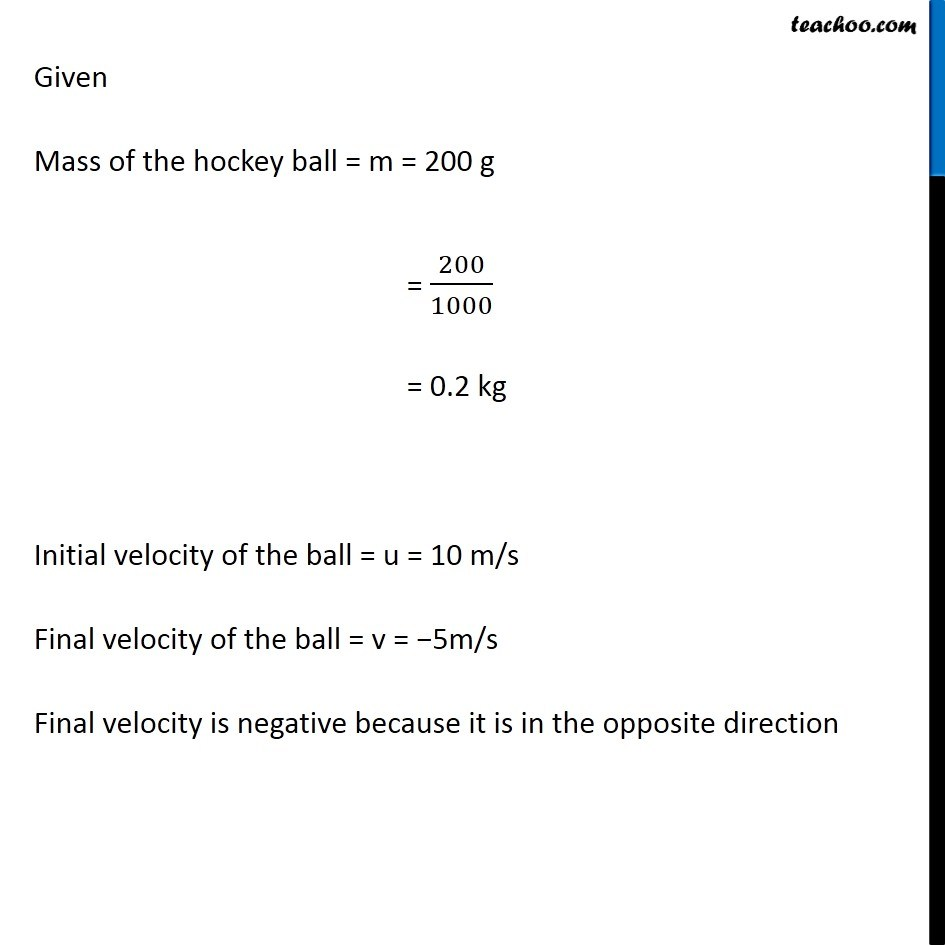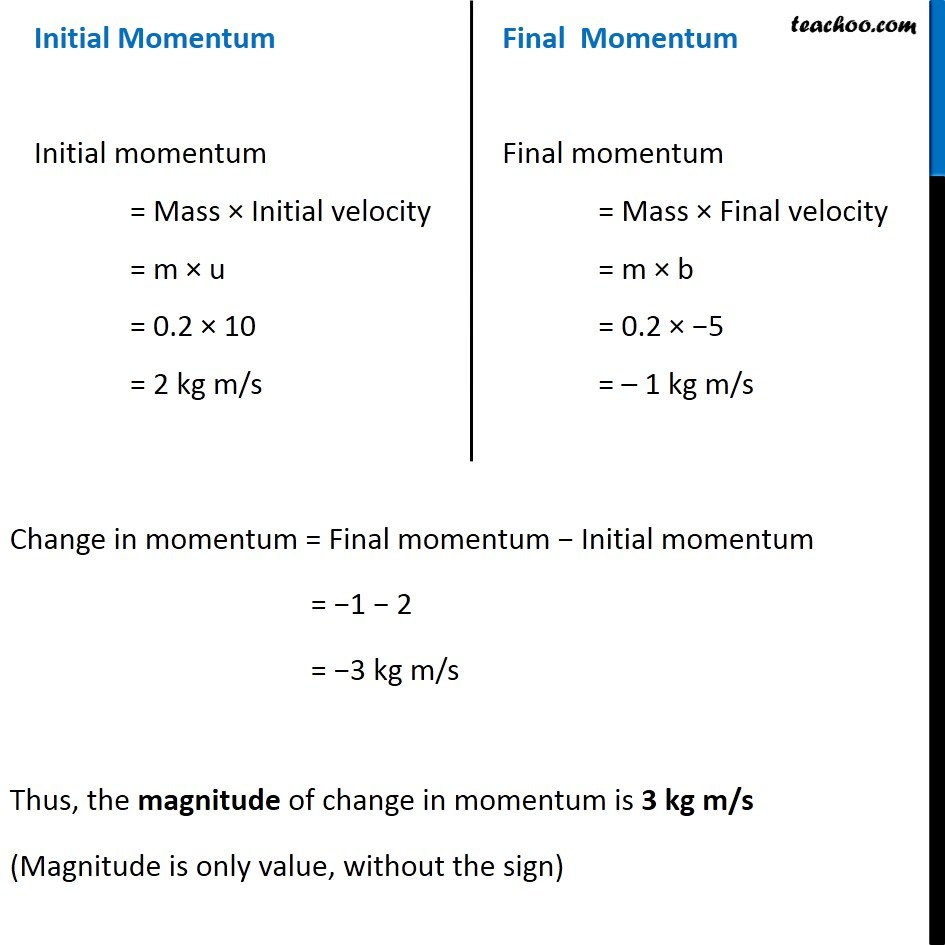NCERT Questions

Class 9
Chapter 9 Class 9 - Force and Laws Of MotionLearn in your speed, with individual attention - Teachoo Maths 1-on-1 Class

### Transcript

NCERT Question 13 A hockey ball of mass 200 g travelling at 10 m s–1 is struck by a hockey stick so as to return it along its original path with a velocity at 5 m s–1. Calculate the magnitude of change of momentum occurred in the motion of the hockey ball by the force applied by the hockey stick. Given Mass of the hockey ball = m = 200 g = 200/1000 = 0.2 kg Initial velocity of the ball = u = 10 m/s Final velocity of the ball = v = −5m/s Final velocity is negative because it is in the opposite direction Initial Momentum Initial momentum = Mass × Initial velocity = m × u = 0.2 × 10 = 2 kg m/s Final Momentum Final momentum = Mass × Final velocity = m × b = 0.2 × −5 = – 1 kg m/s Change in momentum = Final momentum − Initial momentum = −1 − 2 = −3 kg m/s Thus, the magnitude of change in momentum is 3 kg m/s (Magnitude is only value, without the sign)